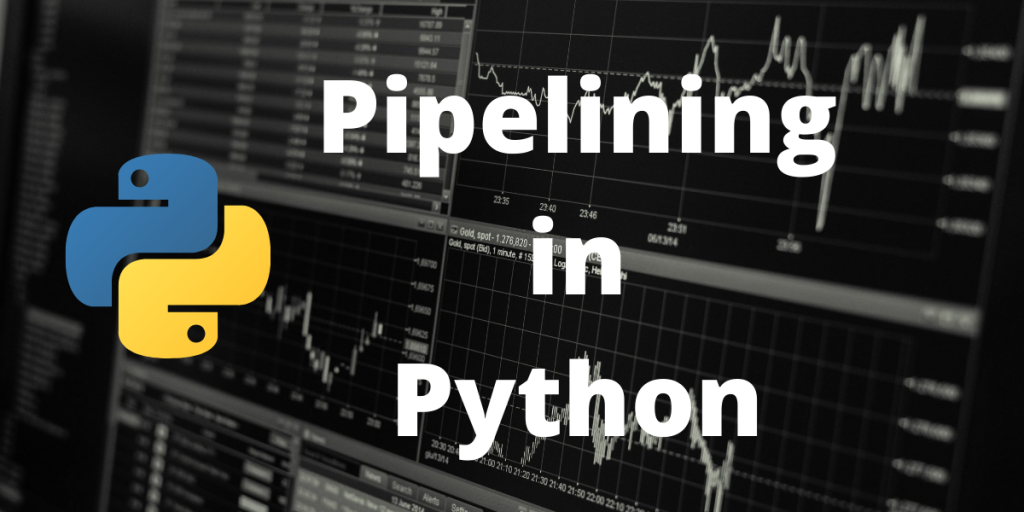# Pipelining in Python – A Complete GuideThis article talks about pipelining in Python. In applied machine learning, there are typical processes. They’re standard because they resolve issues like data leakage in test setups.

The pipeline is a Python scikit-learn utility for orchestrating machine learning operations.

Pipelines function by allowing a linear series of data transforms to be linked together, resulting in a measurable modeling process.

The objective is to guarantee that all phases in the pipeline, such as training datasets or each of the fold involved in the cross-validation technique, are limited to the data available for the assessment.

### Data Preparation and Modeling For Pipelining in Python

The leaking of data from your training dataset to your test dataset is a common pitfall in machine learning and data science.

To prevent falling into this trap, you’ll need a reliable test harness with clear training and testing separation. Data preparation is included.

Data preparation is such a simple approach for the algorithm to acquire access to the entire training dataset. For example, normalizing or standardizing the entire training dataset before learning would not be a proper test because the scale of the data in the test set would have influenced the training dataset.

Pipelines ensure that data preparation, such as normalization, is restricted to each fold of your cross-validation operation, minimizing data leaks in your test harness.

This critical data preparation and model evaluation method is demonstrated in the example below. There are two steps in the pipeline:

• Ensure that the data is uniform.
• Understand how to use a Linear Discriminant Analysis model.

Let’s understand how a pipeline is created in python and how datasets are trained in it.

## Importing Libraries

Creating a pipeline requires lots of import packages to be loaded into the system. Remember, you need to install and configure all these python packages beforehand in order to use them in the program.

```from sklearn.linear_model import LogisticRegression
from sklearn.decomposition import PCA
from sklearn.feature_selection import SelectKBest
from sklearn.model_selection import KFold
from sklearn.model_selection import cross_val_score
from sklearn.pipeline import Pipeline
from sklearn.pipeline import FeatureUnion
```

In this example, will be fetching data from a public domain containing information of people suffering from diabetes. We will be using this database to train our pipeline.

The code below demonstrates how public domain records can be loaded:

```url_data = "https://raw.githubusercontent.com/jbrownlee/Datasets/master/pima-indians-diabetes.data.csv"
varnames = ['var_preg', 'var_plas', 'var_pres', 'var_skin', 'var_test', 'var_mass', 'var_pedi', 'var_age', 'var_class']
vararray = vardataframe.values
varX = vararray[:,0:8]
varY = vararray[:,8]
```

## Creating Pipelines in Python

```urlfeatures = []
urlfeatures.append(('pca', PCA(n_components=3)))
urlfeatures.append(('select_best', SelectKBest(k=6)))
feature_union = FeatureUnion(urlfeatures)
# Here, pipeline is created
estimators = []
estimators.append(('feature_union', feature_union))
estimators.append(('logistic', LogisticRegression()))
model = Pipeline(estimators)
# The pipelie is tested here
seed = 7
varkfold = KFold(n_splits=10)
dataresults = cross_val_score(model, varX, varY, cv=varkfold)
print(dataresults.mean())
```

## Complete Implementation of Pipelining in Python

The whole working program is demonstrated below:

```# Create a pipeline that extracts features from the data then creates a model
from sklearn.linear_model import LogisticRegression
from sklearn.decomposition import PCA
from sklearn.feature_selection import SelectKBest
from sklearn.model_selection import KFold
from sklearn.model_selection import cross_val_score
from sklearn.pipeline import Pipeline
from sklearn.pipeline import FeatureUnion

# data laoded into global variables
url_data = "https://raw.githubusercontent.com/jbrownlee/Datasets/master/pima-indians-diabetes.data.csv"
varnames = ['var_preg', 'var_plas', 'var_pres', 'var_skin', 'var_test', 'var_mass', 'var_pedi', 'var_age', 'var_class']
vararray = vardataframe.values
varX = vararray[:,0:8]
varY = vararray[:,8]

# creating feature union
urlfeatures = []
urlfeatures.append(('pca', PCA(n_components=3)))
urlfeatures.append(('select_best', SelectKBest(k=6)))
feature_union = FeatureUnion(urlfeatures)

# Here, pipeline is created
estimators = []
estimators.append(('feature_union', feature_union))
estimators.append(('logistic', LogisticRegression()))
model = Pipeline(estimators)

# The pipelie is tested here
seed = 7
varkfold = KFold(n_splits=10)
dataresults = cross_val_score(model, varX, varY, cv=varkfold)
print(dataresults.mean())
```

### Output

Let’s look at another example to better understand pipeline testing.

In the code below, an iris database is loaded into the testing pipeline. Iris databases are a classification of databases provided by sklearn to test pipelines. In this example, a single database is used to both train and test the pipeline by splitting it into equal halves, i.e. 50% of the data will be loaded into the testing pipeline while the rest half will be used in the training pipeline.

```from sklearn import datasets
from sklearn.model_selection import train_test_split
from sklearn.preprocessing import StandardScaler
from sklearn.decomposition import PCA
from sklearn.tree import DecisionTreeClassifier
# database is imported from inbuilt sklearn datasets
X = iris.data
y = iris.target

#The data spliting is executed here
X_train, X_test, y_train, y_test = train_test_split(X, y, test_size = 0.50)
# importing pipes for making the Pipe flow
from sklearn.pipeline import Pipeline
# The sequence of pipe flow is :
# PCA dimension is reduced by 2 >> Data gets scaled >> Classification of decission tree
pipe = Pipeline([('pca', PCA(n_components = 2)), ('std', StandardScaler()), ('decision_tree', DecisionTreeClassifier())], verbose = True)

# fitting the data in the pipeline
pipe.fit(X_train, y_train)

# scoring data
from sklearn.metrics import accuracy_score
print(accuracy_score(y_test, pipe.predict(X_test)))
```